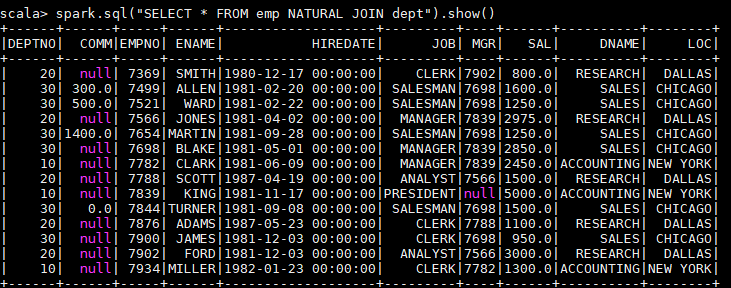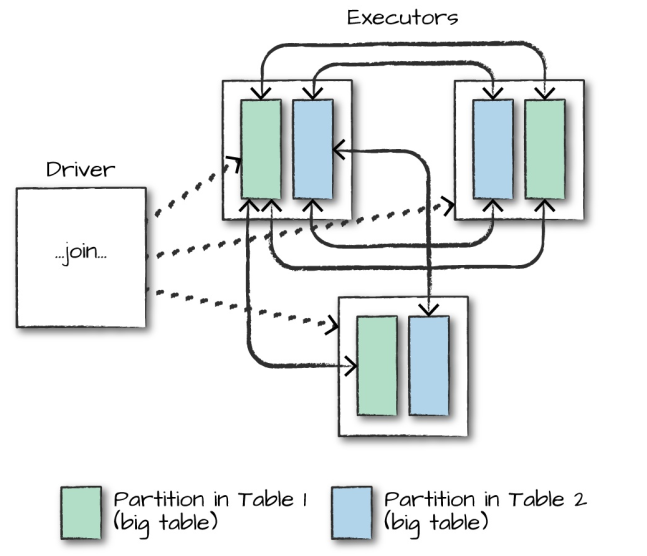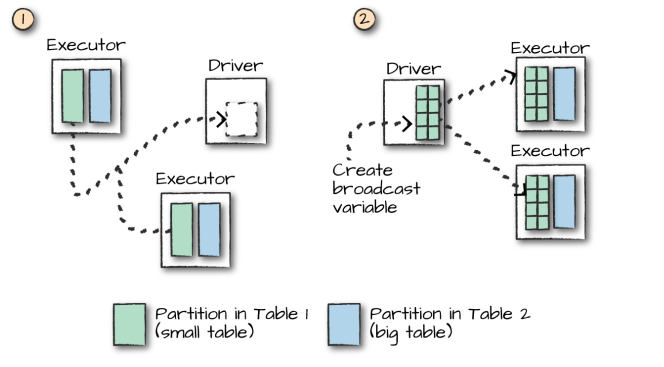# 大数据基础---SparkSQL联结操作

## 一、 数据准备

val spark = SparkSession.builder().appName("aggregations").master("local").getOrCreate()

val empDF = spark.read.json("/usr/file/json/emp.json")
empDF.createOrReplaceTempView("emp")

val deptDF = spark.read.json("/usr/file/json/dept.json")
deptDF.createOrReplaceTempView("dept")


emp 员工表
|-- ENAME: 员工姓名
|-- DEPTNO: 部门编号
|-- EMPNO: 员工编号
|-- HIREDATE: 入职时间
|-- JOB: 职务
|-- MGR: 上级编号
|-- SAL: 薪资
|-- COMM: 奖金

dept 部门表
|-- DEPTNO: 部门编号
|-- DNAME:  部门名称
|-- LOC:    部门所在城市


## 二、连接类型

Spark 中支持多种连接类型：

• Inner Join : 内连接；
• Full Outer Join : 全外连接；
• Left Outer Join : 左外连接；
• Right Outer Join : 右外连接；
• Left Semi Join : 左半连接；
• Left Anti Join : 左反连接；
• Natural Join : 自然连接；
• Cross (or Cartesian) Join : 交叉 (或笛卡尔) 连接。-- LEFT SEMI JOIN
SELECT * FROM emp LEFT SEMI JOIN dept ON emp.deptno = dept.deptno
-- 等价于如下的 IN 语句
SELECT * FROM emp WHERE deptno IN (SELECT deptno FROM dept)

-- LEFT ANTI JOIN
SELECT * FROM emp LEFT ANTI JOIN dept ON emp.deptno = dept.deptno
-- 等价于如下的 IN 语句
SELECT * FROM emp WHERE deptno NOT IN (SELECT deptno FROM dept)


### 2.1 INNER JOIN

// 1.定义连接表达式
val joinExpression = empDF.col("deptno") === deptDF.col("deptno")
// 2.连接查询
empDF.join(deptDF,joinExpression).select("ename","dname").show()

// 等价 SQL 如下：
spark.sql("SELECT ename,dname FROM emp JOIN dept ON emp.deptno = dept.deptno").show()


### 2.2 FULL OUTER JOIN

empDF.join(deptDF, joinExpression, "outer").show()
spark.sql("SELECT * FROM emp FULL OUTER JOIN dept ON emp.deptno = dept.deptno").show()


### 2.3 LEFT OUTER JOIN

empDF.join(deptDF, joinExpression, "left_outer").show()
spark.sql("SELECT * FROM emp LEFT OUTER JOIN dept ON emp.deptno = dept.deptno").show()


### 2.4 RIGHT OUTER JOIN

empDF.join(deptDF, joinExpression, "right_outer").show()
spark.sql("SELECT * FROM emp RIGHT OUTER JOIN dept ON emp.deptno = dept.deptno").show()


### 2.5 LEFT SEMI JOIN

empDF.join(deptDF, joinExpression, "left_semi").show()
spark.sql("SELECT * FROM emp LEFT SEMI JOIN dept ON emp.deptno = dept.deptno").show()


### 2.6 LEFT ANTI JOIN

empDF.join(deptDF, joinExpression, "left_anti").show()
spark.sql("SELECT * FROM emp LEFT ANTI JOIN dept ON emp.deptno = dept.deptno").show()


### 2.7 CROSS JOIN

empDF.join(deptDF, joinExpression, "cross").show()
spark.sql("SELECT * FROM emp CROSS JOIN dept ON emp.deptno = dept.deptno").show()


### 2.8 NATURAL JOIN

spark.sql("SELECT * FROM emp NATURAL JOIN dept").show()


spark.sql("SELECT * FROM emp JOIN dept ON emp.deptno = dept.deptno").show()## 三、连接的执行empDF.join(broadcast(deptDF), joinExpression).show()


## 参考资料

1. Matei Zaharia, Bill Chambers . Spark: The Definitive Guide[M] . 2018-02

posted @ 2020-06-26 17:44  数据驱动  阅读(264)  评论(0编辑  收藏  举报